# FORM ONE MATHEMATICS STUDY NOTES TOPIC 1-3.

3
1531TOPIC 1: NUMBERS

we
know that when we count we start 1,2 …. . But there are other numbers
like 0, negative numbers and decimals. All these types of numbers are
categorized in different groups like counting numbers, integers,real
numbers, whole numbers and rational and irrational numbers according to
their properties. all this have been covered in this chapter
Numbers
are represented by symbols called numerals. For example, numeral for
the number ten is 10. Numeral for the number hundred is 110 and so on.
The
symbols which represent numbers are called digits. For example the
number 521 has three (3) digits which are 5, 2 and 1. There are only
tendigits which are used to represent any number. These digits are 0, 1,
2, 3, 4, 5, 6, 7, 8, and 9.
The Place Value in each Digit in Base Ten Numeration
Identify the place value in each digit in base ten numeration
When
we write a number, for example 521, each digit has a different value
called place value. The 1 on the right means 1 ones which can be written
as 1 × 1, the next number which is 2 means 2 tens which can be written
as 2 × 10 and the last number which is 5 means 5 hundreds which can be
written as 5 × 100. Therefore the number 521 was found by adding the
numbers 5 × 100 + 2 × 10 + 1× 1 = 521.
Note that when writing numbers in words, if there is zero between numbers we use word ‘and’
Example 1
Write the following numbers in words:
1. 7 008
2. 99 827 213
3. 59 000
Solution
1. 7 008 = Seven thousand and eight.
2. 99 827 213 = Ninety nine millions eight hundred twenty seven thousand two hundred thirteen.
3. 59 000 = Fifty nine thousand.
Example 2
Write the numbers bellow in expanded form.
1. 732.
2. 1 205.
Solution
1. 732 = 7 x 100 + 3 x 10 + 2 x 1
2. 1 205 = 1 x 1000 + 2 x 100 + 0 x 10 + 5 x 1
Example 3
Write in numerals for each of the following:
1. 9 x 100 + 8 x 10 + 0 x 1
2. Nine hundred fifty five thousand and five.
Solution
1. 9 x 100 + 8 x 10 + 0 x 1 = 980
2. Nine hundred fifty five thousand and five = 955 005.
Example 4
For each of the following numbers write the place value of the digit in brackets.
1. 89 705 361 (8)
2. 57 341 (7)
Solution
1. 8 is in the place value of ten millions.
2. 7 is in the place value of thousands.
Numbers in Base Ten Numeration
Read numbers in base ten numeration
Base
Ten Numeration is a system of writing numbers using ten symbols i.e. 0,
1, 2, 3, 4, 5, 6, 7, 8 and 9. Base Ten Numeration is also called
decimal system of Numeration.
Numbers in Base Ten Numeration up to One Billion
Write numbers in base ten numeration up to one billion
Consider the table below showing place values of numbers up to one Billion.
 Billions Hundred millions Ten millions Millions Hundred Thousands Ten Thousands Thousands Hundreds Tens Ones 1 1 0 1 0 0 1 0 0 0 1 0 0 0 0 1 0 0 0 0 0 1 0 0 0 0 0 0 1 0 0 0 0 0 0 0 1 0 0 0 0 0 0 0 0 1 0 0 0 0 0 0 0 0 0
If
you are given numerals for a number having more than three digits, you
have to write it by grouping the digits into groups of three digits from
right. For example 7892939 is written as 7 892 939.
When
we are writing numbers in words we consider their place values. For
example; if we are told to write 725 in words, we first need to know the
place value of each digit. Starting from right side 5 is in the place
value of ones, 2 is in the place value of tens and seven is in the place
value of hundreds. Therefore our numeral will be read as seven hundred
twenty five.
Numbers in Daily Life
Apply numbers in daily life
Numbers
play an important role in our lives. Almost all the things we do
involve numbers and Mathematics. Whether we like it or not, our life
revolves in numbers since the day we were born. There are numerous
numbers directly or indirectly connected to our lives.
The following are some uses of numbers in our daily life:
1. Calling a member of a family or a friend using mobile phone.
2. Calculating your daily budget for your food, transportation, and other expenses.
3. Cooking, or anything that involves the idea of proportion and percentage.
4. Weighing fruits, vegetables, meat, chicken, and others in market.
5. Using elevators to go places or floors in the building.
6. Looking at the price of discounted items in a shopping mall.
7. Looking for the number of people who liked your post on Facebook.
8. Switching the channels of your favorite TV shows.
9. Telling time you spent on work or school.
Integers
Identify integers
Consider a number line below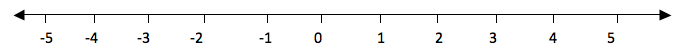The
numbers from 0 to the right are called positive numbers and the numbers
from 0 to the left with minus (-) sign are called negative numbers.
Therefore all numbers with positive (+) or negative (-) sign are called
integers and they are denoted by Ζ. Numbers with positive sign are
written without showing the positive sign. For example +1, +2, +3, …
they are written simply as 1, 2, 3, … . But negative numbers must carry
negative sign (-). Therefore integers are all positive and negative
numbers including zero (0). Zero is neither positive nor negative
number. It is neutral.
The
numbers from zero to the right increases their values as the increase.
While the numbers from zero to the left decrease their values as they
increase. Consider a number line below.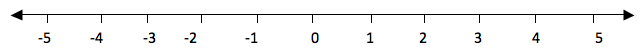If
you take the numbers 2 and 3, 3 is to the right of 2, so 3 is greater
than 2. We use the symbol ‘>’ to show that the number is greater than
i. e. 3 >2(three is greater than two). And since 2 is to the left of
3, we say that 2 is smaller than 3 i.e. 2<3. The symbol ‘<’ is
use to show that the number is less than.
Consider
numbers to the left of 0. For example if you take -5 and -3. -5 is to
the left of -3, therefore -5 is smaller than -3. -3 is to the right of
-5, therefore -3 is greater than -5.
Generally, the number which is to the right of the other number is greater than the number which is to the left of it.
If
two numbers are not equal to each to each other, we use the symbol ‘≠’
to show that the two numbers are not equal. The not equal to ‘≠’ is the
opposite of is equal to ‘=’.
Example 21
Represent the following integers Ζ on a number line
1. 0 is greater than Ζ and Ζ is greater than -4
2. -2 is less than Ζ and Ζ is less than or equal to 1.
Solution
a.
0 is greater than Ζ means the integers to the left of zero and Ζ is
greater than -4 means integers to the left of -4. These numbers are -1,
-2 and -3. Consider number line below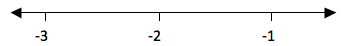b.
-2 is less than Ζ means integers to the right of -2 and Ζ is less than
or equal to 1 means integers to the left of 1 including 1. These
integers are -1, 0 and 1. Consider the number line below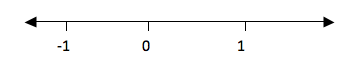Example 22
Put the signs ‘is greater than’ (>), ‘is less than’ (<), ‘is equal to’ (=) to make a true statement.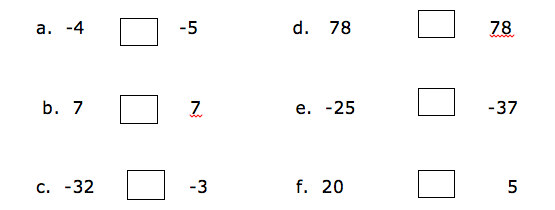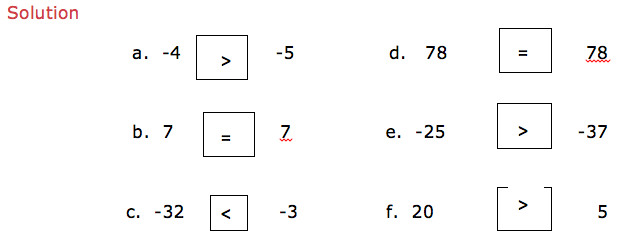Example 23
2 + 3
Show a picture of 2 and 3 on a number line.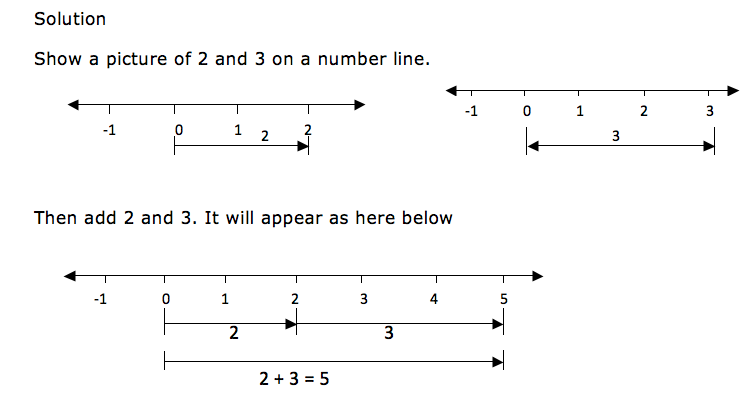When
drawing integers on a number line, the arrows for the positive numbers
goes to the right while the arrows for the negative numbers goes to the
left. Consider an illustration bellow.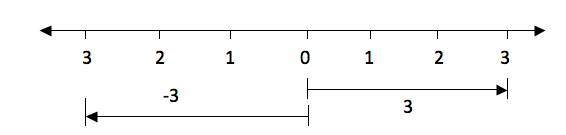The
distance from 0 to 3 is the same as the distance from 0 to -3, only the
directions of their arrows differ. The arrow for positive 3 goes to the
right while the arrow for the negative 3 goes to the left.
Example 24
-3 + 6
Solution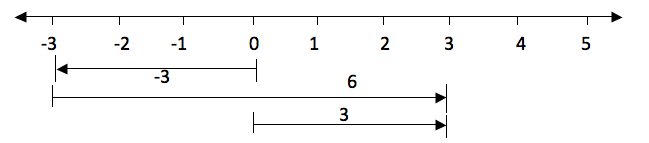Subtraction of Integers
Subtract integers
Since
subtraction is the opposite of addition, if for example you are given
5-4 is the same as 5 + (-4). So if we have to subtract 4 from 5 we can
use a number line in the same way as we did in addition. Therefore 5-4
on a number line will be: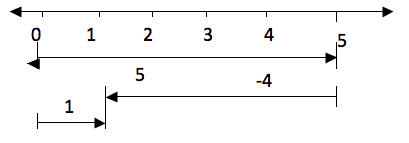Take five steps from 0 to the right and then four steps to the left from 5. The result is 1.
Multiplication of Integers
Multiply integers
Example 25
2×6 is the same as add 2 six times i.e. 2×6 = 2 + 2 + 2 + 2 + 2 +2 = 12. On a number line will be: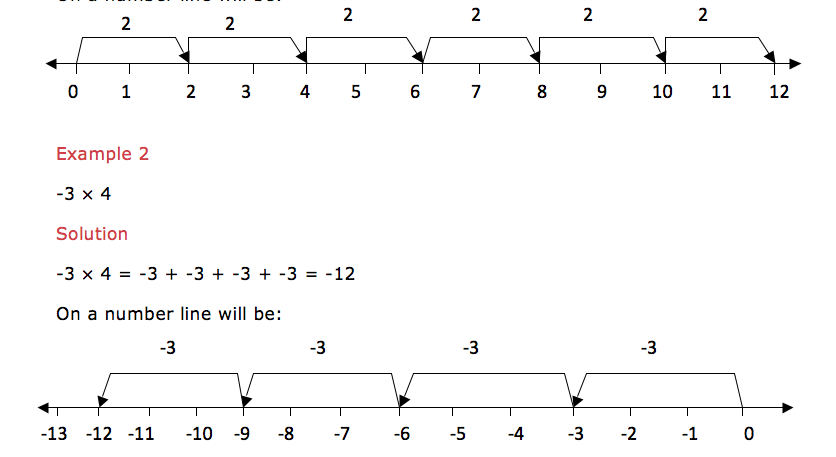Multiplication
of a negative integer by a negative integer cannot be shown on a number
line but the product of these two negative integers is a positive
integer.
From
the above examples we note that multiplication of two positive integers
is a positive integer. And multiplication of a positive integer by a
negative integer is a negative integer. In summary:
• (+)×,(+) = (+)
• (-)×,(-) = (+)
• (+)×,(-) = (-)
• (-)×,(+) = (-)
Division of Integers
Divide integers
Example 26
6÷3 is the same as saying that, which number when you multiply it by 3 you will get 6, that number is 2, so, 6÷3 = 2.
Therefore
division is the opposite of multiplication. From our example 2×3 = 6
and 6÷3 = 2. Thus multiplication and division are opposite to each
other.
Dividing
two integers which are both positive the quotient (answer) is a
positive integer. If they are both negative also the quotient is
positive. If one of the integer is positive and the other is negative
then the quotient is negative. In summary:
• (+)÷(+) = (+)
• (-)÷(-) = (+)
• (+)÷(-) = (-)
• (-)÷(+) = (-)
Mixed Operations on Integers
Perform mixed operations on integers
You
may be given more than one operation on the same problem. Do
multiplication and division first and then the rest of the signs. If
there are brackets, we first open the brackets and then we do division
followed by multiplication, addition and lastly subtraction. In short we
call it BODMAS. The same as the one we did on operations on whole
numbers.
Example 27
9÷3 + 3×2 -1 =
Solution
9÷3 + 3×2 -1
=3 + 6 -1 (first divide and multiply)
Example 28
(12÷4 -2) + 4 – 7=
Solution
(12÷4 -2) + 4 – 7
=1 + 4 – 7 (do operations inside the brackets and divide first)
=2
TOPIC 2: FRACTIONS
A fraction is a number which is expressed in the form of a/b where a – is the top number called numerator and b– is the bottom number called denominator.
A Fraction
Describe a fraction
A fraction is a number which is expressed in the form of a/b where a – is the top number called numerator and b– is the bottom number called denominator.
Consider the diagram below
The shaded part in the diagram above is 1 out of 8, hence mathematically it is written as 1/8Example 1
(a) 3 out of 5 ( three-fifths) = 3/5
Example 2
(b) 7 0ut of 8 ( i.e seven-eighths) = 7/8
Example 3
1. 5/12=(5 X 3)/(12 x 3) =15/36
2. 3/8 =(3 x 2)/(8 X 2) = 6/16
Dividing the numerator and denominator by the same number (This method is used to simplify the fraction)
Difference between Proper, Improper Fractions and Mixed Numbers
Distinguish proper, improper fractions and mixed numbers
Proper fraction –is a fraction in which the numerator is less than denominator
Example 4
4/5, 1/2, 11/13
Improper fraction -is a fraction whose numerator is greater than the denominator
Example 5
12/7, 4/3, 65/56
Mixed fraction –is a fraction which consist of a whole number and a proper fraction
Example 6(a) To convert mixed fractions into improper fractions, use the formula below
(b)To convert improper fractions into mixed fractions, divide the numerator by the denominatorExample 7
Convert the following mixed numbers into improper fractionsOperations on fractions involves addition, subtraction, multiplication and division
• Addition and subtraction of fractions is done by putting both fractions under the same denominator and then add or subtract
• Multiplication
of fractions is done by multiplying the numerator of the first fraction
with the numerator of the second fraction, and the denominator of the
first fraction with the denominator the second fraction.
• For mixed fractions, convert them first into improper fractions and then multiply
• Division of fractions is done by taking the first fraction and then multiply with the reciprocal of the second fraction
• For mixed fractions, convert them first into improper fractions and then divide
Example 11
Find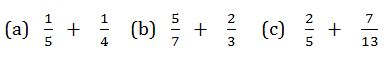Solution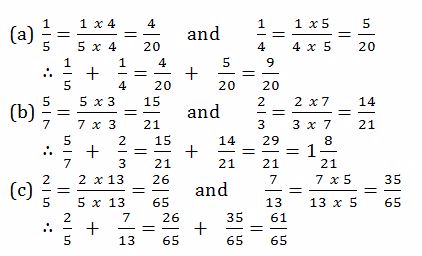Subtraction of Fractions
Subtract fractions
Example 12
Evaluate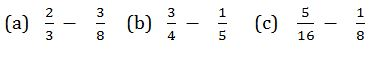Solution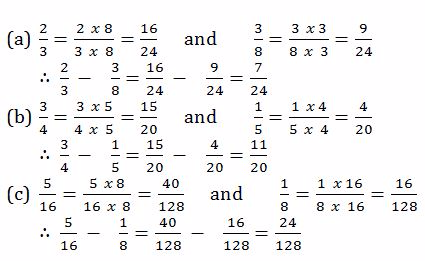Multiplication of Fractions
Multiply fractions
Example 13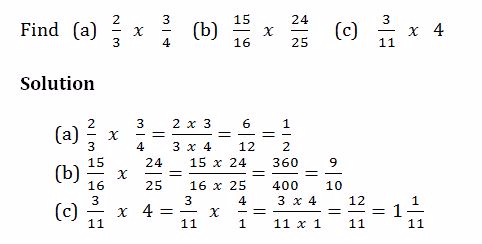Division of Fractions
Divide fractions
Example 14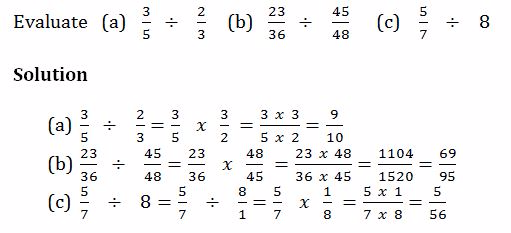Mixed Operations on Fractions
Perform mixed operations on fractions
Example 15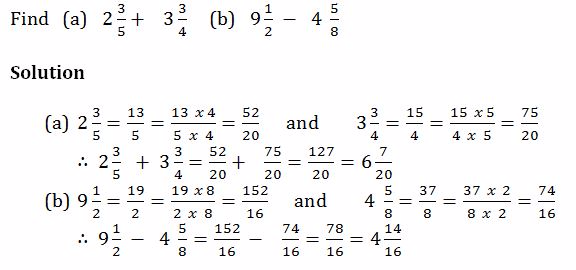Example 16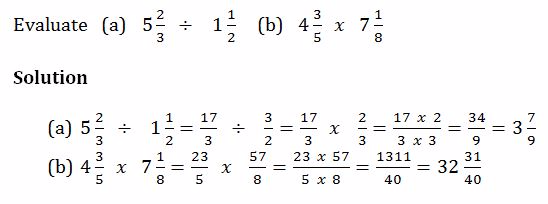Word Problems Involving Fractions
Solve word problems involving fractions
Example 17
1. Musa is years old. His father is 3¾times as old as he is. How old is his father?
2. 1¾of a material are needed to make suit. How many suits can be made from
TOPIC 3: DECIMAL AND PERCENTAGE

The Concept of Decimals
Explain the concept of decimals
A
decimal- is defined as a number which consist of two parts separated by
a point.The parts are whole number part and fractional part
Example 1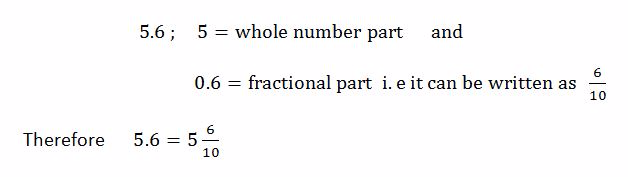Example 2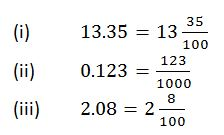Conversion of Fractions to Terminating Decimals and Vice Versa
Convert fractions to terminating decimals and vice versa
The first place after the decimal point is called tenths.The second place after the decimal point is called hundredths e.t.c
Consider the decimal number 8.152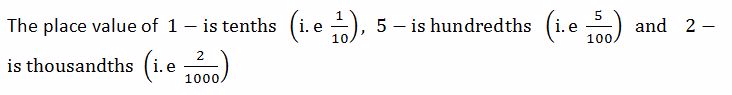NOTE
• To convert a fraction into decimal, divide the numerator by denominator
• To
convert a decimal into fraction, write the digits after the decimal
point as tenths, or hundredths or thousandths depending on the number of
decimal places.
Example 3
Convert the following fractions into decimals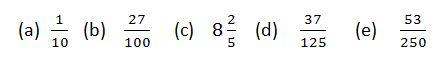Solution
Divide the numerator by denominator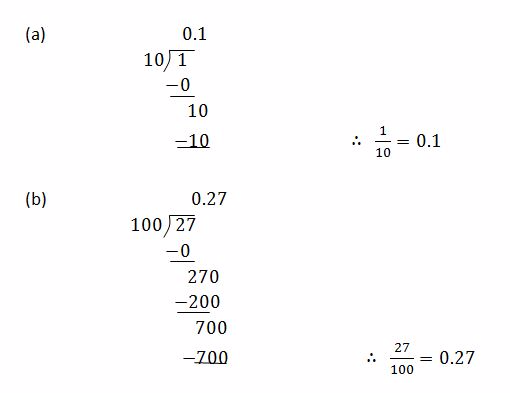2.dj_mafuvu.blogspot.com
3.TEACHERLOOKUP. COM